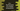# C++ program to find the factorial of a number using pointer# C++ program to find factorial using pointer :

In this tutorial, we will learn how to find the factorial of a number using pointers. This program will take one number as input from the user, calculate the factorial and print it out.

We are going to use one recursive function to find out the factorial and one pointer variable to store the address of the user input variable.

## C++ program :

``````#include <iostream>
using namespace std;

int findFactorial(int no)
{
if (no == 2)
{
return no;
}

return no * findFactorial(no - 1);
}

int main()
{
int *ptr;
int no;

cout << "Enter a number to find factorial : " << endl; cin >> no;

ptr = &no;
cout << findFactorial(*ptr) << endl;
}``````

### Explanation :

1. findFactorial method takes one integer value as its parameter. If the value of this parameter is 2, it returns the same value. Else, it returns the multiplication of no and factorial of no - 1, where no is the parameter.
2. We have one integer pointer variable ptr. It can store the address of an integer variable.
3. The program asks the user to enter a number. It will read and store it in the integer variable no.
4. Assign the address of the variable no to the pointer variable ptr
5. Call the findFactorial method to calculate the factorial. *ptr is used to get the value stored in this address of the pointer variable.

### Sample output :

``````Enter a number to find factorial :
10
3628800

Enter a number to find factorial :
5
120

Enter a number to find factorial :
13
1932053504``````